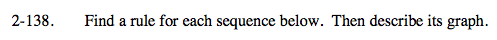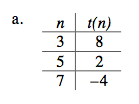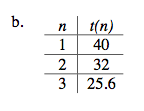### Home > A2C > Chapter 2 > Lesson 2.2.2 > Problem2-138

2-138.First, decide if the given sequence is arithmetic or geometric.

Common difference =

$\frac{\Delta t(n)}{\Delta n} = \frac{2 -8 }{5 -3} = \frac{-6}{2}=-3$

So, t(n) = −3n + b.

Find the value of b at (3, 8).

Write the equation once you know b. Then describe the graph.

t(n) = −3n + 17

The graph is a straight line with the y-intercept at (0, 17) and a slope of −3.The multiplier is less than 1.

You will need to find t(0) to write the equation.

This graph is not linear.

Use the eTool below to view the graphs.
Click the link at right for the full version of the eTool: A2C 2-138 HW eTool